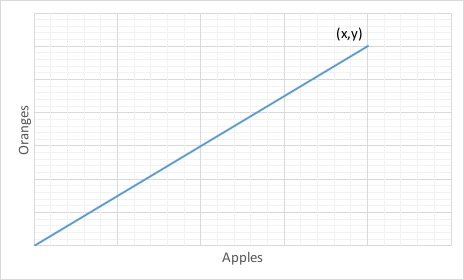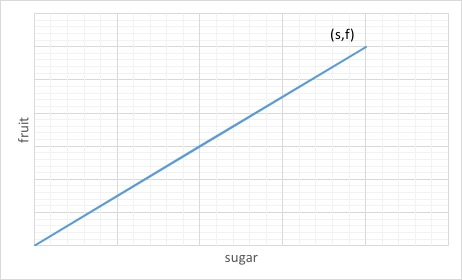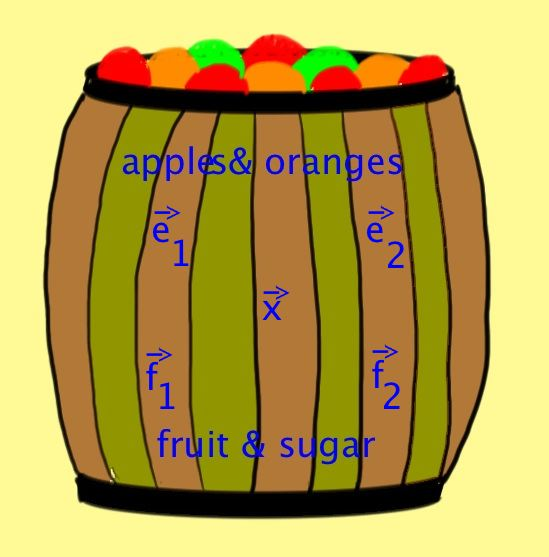$$\newcommand{\id}{\mathrm{id}}$$ $$\newcommand{\Span}{\mathrm{span}}$$ $$\newcommand{\kernel}{\mathrm{null}\,}$$ $$\newcommand{\range}{\mathrm{range}\,}$$ $$\newcommand{\RealPart}{\mathrm{Re}}$$ $$\newcommand{\ImaginaryPart}{\mathrm{Im}}$$ $$\newcommand{\Argument}{\mathrm{Arg}}$$ $$\newcommand{\norm}{\| #1 \|}$$ $$\newcommand{\inner}{\langle #1, #2 \rangle}$$ $$\newcommand{\Span}{\mathrm{span}}$$

# 18.12: Movie Scripts 11-12

$$\newcommand{\vecs}{\overset { \rightharpoonup} {\mathbf{#1}} }$$ $$\newcommand{\vecd}{\overset{-\!-\!\rightharpoonup}{\vphantom{a}\smash {#1}}}$$$$\newcommand{\id}{\mathrm{id}}$$ $$\newcommand{\Span}{\mathrm{span}}$$ $$\newcommand{\kernel}{\mathrm{null}\,}$$ $$\newcommand{\range}{\mathrm{range}\,}$$ $$\newcommand{\RealPart}{\mathrm{Re}}$$ $$\newcommand{\ImaginaryPart}{\mathrm{Im}}$$ $$\newcommand{\Argument}{\mathrm{Arg}}$$ $$\newcommand{\norm}{\| #1 \|}$$ $$\newcommand{\inner}{\langle #1, #2 \rangle}$$ $$\newcommand{\Span}{\mathrm{span}}$$ $$\newcommand{\id}{\mathrm{id}}$$ $$\newcommand{\Span}{\mathrm{span}}$$ $$\newcommand{\kernel}{\mathrm{null}\,}$$ $$\newcommand{\range}{\mathrm{range}\,}$$ $$\newcommand{\RealPart}{\mathrm{Re}}$$ $$\newcommand{\ImaginaryPart}{\mathrm{Im}}$$ $$\newcommand{\Argument}{\mathrm{Arg}}$$ $$\newcommand{\norm}{\| #1 \|}$$ $$\newcommand{\inner}{\langle #1, #2 \rangle}$$ $$\newcommand{\Span}{\mathrm{span}}$$

## G.11 Eigenvalues and Eigenvectors

$$2\times 2$$ Example

Here is an example of how to find the eigenvalues and eigenvectors of a $$2 \times 2$$ matrix.

$M =\begin{pmatrix}4 & 2\\1 & 3\\\end{pmatrix}.$

Remember that an eigenvector $$v$$ with eigenvalue $$\lambda$$ for $$M$$ will be a vector such that $$Mv = \lambda v$$ i.e. $$M(v) - \lambda I (v) = \vec{0}$$. When we are talking about a nonzero $$v$$ then this means that $$\det (M - \lambda I) = 0$$. We will start by finding the eigenvalues that make this statement true. First we compute

$\det (M - \lambda I) = \det \left(\begin{pmatrix}4 & 2\\1 & 3\\\end{pmatrix} - \begin{pmatrix}\lambda & 0\\0 & \lambda\\ \end{pmatrix} \right) = \det \begin{pmatrix}4-\lambda & 2\\1 & 3-\lambda \\\end{pmatrix}$

so $$\det (M - \lambda I)= (4-\lambda)(3-\lambda ) - 2\cdot1$$. We set this equal to zero to find values of $$\lambda$$ that make this true:

$(4-\lambda)(3-\lambda ) - 2\cdot1 = 10-7\lambda +\lambda^2 = (2-\lambda)(5-\lambda) = 0\, .$

This means that $$\lambda= 2$$ and $$\lambda= 5$$ are solutions. Now if we want to find the eigenvectors that correspond to these values we look at vectors $$v$$ such that

$\begin{pmatrix}4-\lambda & 2\\1 & 3-\lambda \\\end{pmatrix} v = \vec 0 \, .$

For $$\lambda= 5$$

$\begin{pmatrix}4-5 & 2\\1 & 3-5 \\\end{pmatrix} \begin{pmatrix}x\\y\end{pmatrix} = \begin{pmatrix}-1 & 2\\1 & -2 \\\end{pmatrix} \begin{pmatrix}x\\y\end{pmatrix} = \vec 0 \, .$

This gives us the equalities $$-x +2y = 0$$ and $$x -2y = 0$$ which both give the line $$y = \frac{1}{2}x$$. Any point on this line, so for example $$\begin{pmatrix}2\\1\end{pmatrix}$$, is an eigenvector with eigenvalue $$\lambda = 5$$.

Now lets find the eigenvector for $$\lambda = 2$$

$\begin{pmatrix}4-2 & 2\\1 & 3-2 \\\end{pmatrix} \begin{pmatrix}x\\y\end{pmatrix} = \begin{pmatrix}2 & 2\\1 & 1 \\\end{pmatrix} \begin{pmatrix}x\\y\end{pmatrix} = \vec 0 ,$

which gives the equalities $$2x+2y = 0$$ and $$x+y = 0$$.

(Notice that these equations are not independent of one another, so our eigenvalue must be correct.)

This means any vector $$v = \begin{pmatrix}x\\y\end{pmatrix} where \(y = -x$$ , such as $$\begin{pmatrix}1\\-1\end{pmatrix}, or any scalar multiple of this vector, \(\textit{i.e.}$$ any vector on the line $$y = -x$$ is an eigenvector with eigenvalue 2. This solution could be written neatly as

$\lambda_{1} = 5, \, v_{1}= \begin{pmatrix}2\\1\end{pmatrix} \, \text{ and } \lambda_{2} = 2, \, v_{2}=\begin{pmatrix}1\\-1\end{pmatrix}.$

Jordan Block Example

Consider the matrix

$J_{2} = \begin{pmatrix}\lambda & 1\\ 0 & \lambda\end{pmatrix},$

and we note that we can just read off the eigenvector $$e_{1}$$ with eigenvalue $$\lambda$$. However the characteristic polynomial of $$J_{2}$$ is $$P_{J_{2}}(\mu) = (\mu - \lambda)^{2}$$ so the only possible eigenvalue is $$\lambda$$, but we claim it does not have a second eigenvector $$v$$. To see this, we require that

\begin{align*}\lambda v^{1} + v^{2} & = \lambda v^{1}\\ \lambda v^{2} & = \lambda v^{2}\end{align*}

which clearly implies that $$v^{2} = 0$$. This is known as a Jordan $$2$$-cell, and in general, a Jordan $$n$$-cell with eigenvalue $$\lambda$$ is (similar to) the $$n \times n$$ matrix

$J_{n} = \begin{pmatrix}\lambda & 1 & 0 & \cdots & 0 \\0 & \lambda & 1 & \ddots & 0 \\\vdots & \ddots & \ddots & \ddots & \vdots \\0 & \cdots & 0 & \lambda & 1 \\0 & \cdots & 0 & 0 & \lambda\end{pmatrix}$

which has a single eigenvector $$e_{1}$$.

Now consider the following matrix

$M = \begin{pmatrix}3 & 1 & 0 \\0 & 3 & 1 \\0 & 0 & 2\end{pmatrix}$

and we see that $$P_{M}(\lambda) = (\lambda - 3)^{2}(\lambda - 2)$$. Therefore for $$\lambda = 3$$ we need to find the solutions to $$(M - 3I_{3})v = 0$$ or in equation form:

\begin{align*}v^{2} & = 0\\ v^{3} & = 0\\ -v^{3} & = 0,\end{align*}

and we immediately see that we must have $$V = e_{1}$$. Next for $$\lambda = 2$$, we need to solve $$(M - 2I_{3})v = 0$$ or

\begin{align*}v^{1} + v^{2} & = 0\\ v^{2} + v^{3} & = 0\\ 0 & = 0,\end{align*}

and thus we choose $$v^{1} = 1$$, which implies $$v^{2} = -1$$ and $$v^{3} = 1$$. Hence this is the only other eigenvector for $$M$$.

$$\textit{This is a specific case of Problem 13.7.}$$

Eigenvalues

Eigenvalues and eigenvectors are extremely important. In this video we review the theory of eigenvalues. Consider a linear transformation

$L:V\longrightarrow V$

where $$\dim V=n<\infty$$. Since $$V$$ is finite dimensional, we can represent $$L$$ by a square matrix $$M$$ by choosing a basis for $$V$$.

So the eigenvalue equation

$Lv=\lambda v$

becomes

$M v = \lambda v$

where $$v$$ is a column vector and $$M$$ is an $$n\times n$$ matrix (both expressed in whatever basis we chose for $$V$$). The scalar $$\lambda$$ is called an eigenvalue of $$M$$ and the job of this video is to show you how to find all the eigenvalues of $$M$$.

The first step is to put all terms on the left hand side of the equation, this gives

$(M-\lambda I) v = 0\, .$

Notice how we used the identity matrix $$I$$ in order to get a matrix times $$v$$ equaling zero. Now here comes a VERY important fact

$N u = 0 and u\neq 0 \Longleftrightarrow \det N=0.$

$$\textit{I.e., a square matrix can have an eigenvector with vanishing eigenvalue if and only if its determinant vanishes!}$$ Hence

$\det(M-\lambda I) = 0.$

The quantity on the left (up to a possible minus sign) equals the so-called characteristic polynomial

$P_{M}(\lambda):=\det(\lambda I - M)\, .$

It is a polynomial of degree $$n$$ in the variable $$\lambda$$. To see why, try a simple $$2\times 2$$ example

$\det\left(\begin{pmatrix}a & b\\c & d\end{pmatrix}-\begin{pmatrix}\lambda & 0\\0 & \lambda\end{pmatrix}\right)=\det\begin{pmatrix}a-\lambda & b\\c & d-\lambda\end{pmatrix}=(a-\lambda)(d-\lambda)-bc\, ,$

which is clearly a polynomial of order $$2$$ in $$\lambda$$. For the $$n\times n$$ case, the order $$n$$ term comes from the product of diagonal matrix elements also.

There is an amazing fact about polynomials called the $$\textit{fundamental theorem of algebra}$$: they can always be factored over complex numbers. This means that degree $$n$$ polynomials have $$n$$ complex roots (counted with multiplicity). The word can does not mean that explicit formulas for this are known (in fact explicit formulas can only be give for degree four or less). The necessity for complex numbers is easily seems from a polynomial like

$z^{2}+1$

whose roots would require us to solve $$z^{2}=-1$$ which is impossible for real number $$z$$. However, introducing the imaginary unit $$i$$ with

$i^{2}=-1\, ,$

we have

$z^{2}+1=(z-i)(z+i)\, .$

Returning to our characteristic polynomial, we call on the fundamental theorem of algebra to write

$P_{M}(\lambda)=(\lambda-\lambda_{1})(\lambda-\lambda_{2})\cdots (\lambda-\lambda_{n})\, .$

The roots $$\lambda_{1}$$, $$\lambda_{2}$$,...,$$\lambda_{n}$$ are the eigenvalues of $$M$$ (or its underlying linear transformation $$L$$).

Eigenspaces

Consider the linear map

$L = \begin{pmatrix}-4 & 6 & 6 \\0 & 2 & 0 \\-3 & 3 &5\end{pmatrix}.$

Direct computation will show that we have

$L = Q \begin{pmatrix}-1 & 0 & 0 \\0 & 2 & 0 \\0 & 0 & 2\end{pmatrix} Q^{-1}$

where

$Q = \begin{pmatrix}2 & 1 & 1 \\0 & 0 & 1 \\1 & 1 & 0\end{pmatrix}.$

Therefore the vectors

$v_{1}^{(2)} = \begin{pmatrix}1 \\ 0 \\ 1\end{pmatrix} \hspace{20pt} v_{2}^{(2)} = \begin{pmatrix}1 \\ 1 \\ 0\end{pmatrix}$

span the eigenspace $$E^{(2)}$$ of the eigenvalue 2, and for an explicit example, if we take

$v = 2 v_{1}^{(2)} - v_{2}^{(2)} = \begin{pmatrix} 1 \\ -1 \\ 2\end{pmatrix}$

we have

$L v = \begin{pmatrix} 2 \\ -2 \\ 4\end{pmatrix} = 2 v$

so $$v \in E^{(2)}$$. In general, we note the linearly independent vectors $$v_{i}^{(\lambda)}$$ with the same eigenvalue $$\lambda$$ span an eigenspace since for any $$v = \sum_{i} c^{i} v_{i}^{(\lambda)}$$, we have

$Lv = \sum_{i} c^{i} Lv_{i}^{(\lambda)} = \sum_{i} c^{i} \lambda v_{i}^{(\lambda)} = \lambda \sum_{i} c^{i} v_{i}^{(\lambda)} = \lambda v.$

Hint for Review Problem 9

We are looking at the matrix $$M$$, and a sequence of vectors starting with $$v(0) = \begin{pmatrix}x(0) \\y(0)\end{pmatrix}$$ and defined recursively so that

$v(1) = \begin{pmatrix}x(1) \\y(1)\end{pmatrix} = M\begin{pmatrix}x(0) \\y(0)\end{pmatrix}.$ We first examine the eigenvectors and eigenvalues of

$M=\begin{pmatrix}3 & 2 \\2 &3 \\\end{pmatrix}.$

We can find the eigenvalues and vectors by solving $\det (M - \lambda I) = 0$ for $$\lambda$$.

$\det \begin{pmatrix}3 -\lambda & 2 \\2 & 3 -\lambda \\\end{pmatrix} = 0$

By computing the determinant and solving for $$\lambda$$ we can find the eigenvalues $$\lambda = 1$$ and $$5$$, and the corresponding eigenvectors. You should do the computations to find these for yourself.

When we think about the question in part (b) which asks to find a vector $$v(0)$$ such that $$v(0) = v(1) =v(2) \ldots$$, we must look for a vector that satisfies $$v = M v$$. What eigenvalue does this correspond to? If you found a $$v(0)$$ with this property would $$c v(0)$$ for a scalar $$c$$ also work? Remember that eigenvectors have to be nonzero, so what if $$c=0$$?

For part (c) if we tried an eigenvector would we have restrictions on what the eigenvalue should be? Think about what it means to be pointed in the same direction.

## G.12 Diagonalization

Non Diagonalizable Example

First recall that the derivative operator is linear and that we can write it as the matrix

$\frac{d}{dx} =\begin{pmatrix}0 & 1 & 0 & 0 & \cdots \\0 & 0 & 2 & 0 & \cdots \\0 & 0 & 0 & 3 & \cdots \\\vdots & \vdots & \vdots & \vdots & \ddots\end{pmatrix}.$

We note that this transforms into an infinite Jordan cell with eigenvalue 0 or

$\begin{pmatrix}0 & 1 & 0 & 0 & \cdots \\0 & 0 & 1 & 0 & \cdots \\0 & 0 & 0 & 1 & \cdots \\\vdots & \vdots & \vdots & \vdots & \ddots\end{pmatrix}$

which is in the basis $$\{n^{-1} x^{n} \}_{n}$$ (where for $$n = 0$$, we just have 1). Therefore we note that $$1$$ (constant polynomials) is the only eigenvector with eigenvalue $$0$$ for polynomials since they have finite degree, and so the derivative is not diagonalizable. Note that we are ignoring infinite cases for simplicity, but if you want to consider infinite terms such as convergent series or all formal power series where there is no conditions on convergence, there are many eigenvectors. Can you find some? This is an example of how things can change in infinite dimensional spaces.

For a more finite example, consider the space $$\mathbb{P}^{\mathbb{C}}_{3}$$ of complex polynomials of degree at most 3, and recall that the derivative $$D$$ can be written as

$D = \begin{pmatrix}0 & 1 & 0 & 0 \\0 & 0 & 2 & 0 \\0 & 0 & 0 & 3 \\0 & 0 & 0 & 0\end{pmatrix}.$

You can easily check that the only eigenvector is $$1$$ with eigenvalue $$0$$ since $$D$$ always lowers the degree of a polynomial by 1 each time it is applied. Note that this is a nilpotent matrix since $$D^{4} = 0$$, but the only nilpotent matrix that is “diagonalizable'' is the $$0$$ matrix.

Change of Basis Example

This video returns to the example of a barrel filled with fruitas a demonstration of changing basis.

Since this was a linear systems problem, we can try to represent what's in the barrel using a vector space. The first representation was the one where $$(x,y)=(\mbox{apples},\mbox{oranges})$$:Calling the basis vectors $$\vec e_{1}:=(1,0)$$ and $$\vec e_{2}:=(0,1)$$, this representation would label what's in the barrel by a vector

$\vec x: = x \vec e_{1} + y\vec e_{2} = \begin{pmatrix}\vec e_{1} & \vec e_{2}\end{pmatrix}\begin{pmatrix}x \\ y\end{pmatrix}\, .$

Since this is the method ordinary people would use, we will call this the “engineer's'' method!

But this is not the approach nutritionists would use. They would note the amount of sugar and total number of fruit $$(s,f)$$:WARNING: To make sense of what comes next you need to allow for the possibity of a negative amount of fruit or sugar. This would be just like a bank, where if money is owed to somebody else, we can use a minus sign.

The vector $$\vec x$$ says what is in the barrel and does not depend which mathematical description is employed. The way nutritionists label $$\vec x$$ is in terms of a pair of basis vectors $$\vec f_{1}$$ and $$\vec f_{2}$$:

$\vec x=s \vec f_{1} + f \vec f_{2}=\begin{pmatrix}\vec f_{1} & \vec f_{2}\end{pmatrix}\begin{pmatrix}s \\ f\end{pmatrix}\, .$

Thus our vector space now has a bunch of interesting vectors:The vector $$\vec x$$ labels generally the contents of the barrel. The vector $$\vec e_{1}$$ corresponds to one apple and one orange. The vector $$\vec e_{2}$$ is one orange and no apples. The vector $$\vec f_{1}$$ means one unit of sugar and zero total fruit (to achieve this you could lend out some apples and keep a few oranges). Finally the vector $$\vec f_{2}$$ represents a total of one piece of fruit and no sugar.

You might remember that the amount of sugar in an apple is called $$\lambda$$ while oranges have twice as much sugar as apples. Thus

$\left\{\begin{matrix}s=\lambda (x+2y) \\ f=x+y \end{matrix}\right.$

Essentially, this is already our change of basis formula, but lets play around and put it in our notations. First we can write this as a matrix

$\begin{pmatrix}s\\f\end{pmatrix} = \begin{pmatrix}\lambda & 2\lambda \\1 & 1\end{pmatrix}\begin{pmatrix}x\\y\end{pmatrix}\, .$

We can easily invert this to get

$\begin{pmatrix}x\\y\end{pmatrix} = \begin{pmatrix}-\frac{1}{\lambda} & 2\\ \frac{1}{\lambda} & -1\end{pmatrix}\begin{pmatrix}s\\f\end{pmatrix}\, .$

Putting this in the engineer's formula for $$\vec x$$ gives

$\vec x = \begin{pmatrix}\vec e_{1} & \vec e_{2}\end{pmatrix}\begin{pmatrix}-\frac{1}{\lambda} & 2\\ \frac{1}{\lambda} & -1\end{pmatrix}\begin{pmatrix}s\\f\end{pmatrix} = \begin{pmatrix}-\frac{1}{\lambda}\big(\vec e_{1} - \vec e_{2}\big) &2\vec e_{1}-2\vec e_{2}\end{pmatrix}\begin{pmatrix}s\\f\end{pmatrix}\, .$

Comparing to the nutritionist's formula for the same object $$\vec x$$ we learn that

$\vec f_{1} = -\frac{1}{\lambda}\big(\vec e_{1} - \vec e_{2}\big)\quad \mbox{and}\quad\vec f_{2}=2\vec e_{1}-2\vec e_{2}\, .$

Rearranging these equation we find the change of base matrix $$P$$ from the engineer's basis to the nutritionist's basis:

$\begin{pmatrix}\vec f_{1} & \vec f_{2}\end{pmatrix} = \begin{pmatrix}\vec e_{1} & \vec e_{2}\end{pmatrix}\begin{pmatrix}-\frac{1}{\lambda} & 2\\ \frac{1}{\lambda} & -1\end{pmatrix} =:\begin{pmatrix}\vec e_{1} & \vec e_{2}\end{pmatrix} \, P\, .$

We can also go the other direction, changing from the nutritionist's basis to the engineer's basis

$\begin{pmatrix}\vec e_{1} & \vec e_{2}\end{pmatrix} = \begin{pmatrix}\vec f_{1} & \vec f_{2}\end{pmatrix}\begin{pmatrix}\lambda & 2\lambda \\ 1 & 1\end{pmatrix} =:\begin{pmatrix}\vec f_{1} & \vec f_{2}\end{pmatrix} \, Q\, .$

Of course, we must have

$Q=P^{-1}\, ,$

(which is in fact how we constructed $$P$$ in the first place).

Finally, lets consider the very first linear systems problem, where you were given that there were 27 pieces of fruit in total and twice as many oranges as apples. In equations this says just

$x+y=27\quad \mbox{and} \quad 2x-y=0\, .$

But we can also write this as a matrix system

$MX=V$

where

$M:=\begin{pmatrix} 1 & 1 \\ 2 & -1 \end{pmatrix}\, , X:=\begin{pmatrix}x \\y \end{pmatrix}\, , V:=\begin{pmatrix}0 \\ 27\end{pmatrix}\, .$

Note that

$\vec x = \begin{pmatrix}\vec e_{1} & \vec e_{2}\end{pmatrix}\, X\, .$

Also lets call  \vec v:= \begin{pmatrix}\vec e_{1} & \vec e_{2}\end{pmatrix}\, V\, .\]

Now the matrix $$M$$ is the matrix of some linear transformation $$L$$ in the basis of the engineers.

Lets convert it to the basis of the nutritionists:

$L\vec x = L \begin{pmatrix}\vec f_{1} & \vec f_{2}\end{pmatrix}\begin{pmatrix} s \\ f\end{pmatrix} = L \begin{pmatrix}\vec e_{1} & \vec e_{2}\end{pmatrix} P \begin{pmatrix} s \\ f\end{pmatrix} = \begin{pmatrix} \vec e_{1} \\ \vec e_{2}\end{pmatrix} M P \begin{pmatrix} s \\ f\end{pmatrix}\, .$

Note here that the linear transformation on acts on {\it vectors} -- these are the objects we have written with a $$\vec{}$$ sign on top of them. It does not act on columns of numbers!

We can easily compute $$MP$$ and find

$MP=\begin{pmatrix} 1 & 1 \\ 2 & -1 \end{pmatrix}\begin{pmatrix}-\frac{1}{\lambda} & 2\\ \frac{1}{\lambda} & -1\end{pmatrix} = \begin{pmatrix}0& 1\\ -\frac{3}{\lambda} & 5\end{pmatrix}\, .$

Note that $$P^{-1}MP$$ is the matrix of $$L$$ in the nutritionists basis, but we don't need this quantity right now.

Thus the last task is to solve the system, lets solve for sugar and fruit.

We need to solve

$MP\begin{pmatrix} s \\ f\end{pmatrix} = \begin{pmatrix}0& 1\\ -\frac{3}{\lambda} & 5\end{pmatrix}\begin{pmatrix} s \\ f\end{pmatrix} = \begin{pmatrix}27\\0\end{pmatrix}\, .$

This is solved immediately by forward substitution (the nutritionists basis is nice since it directly gives $f$):

$f=27\quad \mbox{and} \quad s= 45\lambda\, .$

$$2\times2$$ Example

Lets diagonalize the matrix M from a previous example Eigenvalues and Eigenvectors: $$2\times 2$$ Example

$M = \begin{pmatrix}4 & 2\\1 &3\end{pmatrix}$

We found the eigenvalues and eigenvectors of $$M$$, our solution was

$\lambda_{1} = 5, \, \mathbf{v}_{1}= \begin{pmatrix}2\\1\end{pmatrix} \, \text{ and } \lambda_{2} = 2, \, \mathbf{v}_{2}=\begin{pmatrix}1\\-1\end{pmatrix}.$

So we can diagonalize this matrix using the formula $$D = P^{-1}MP$$ where $$P= (\mathbf{v}_{1}, \mathbf{v}_{2})$$. This means

$P = \begin{pmatrix}2 &1\\1 &-1\end{pmatrix} \text{ and } P^{-1} = -\frac{1}{3}\begin{pmatrix}1 &1\\1 &-2\end{pmatrix}$

The inverse comes from the formula for inverses of $$2\times2$$ matrices:

$\begin{pmatrix}a & b \\c & d \\\end{pmatrix}^{-1}=\frac{1}{ad-bc}\begin{pmatrix}d & -b \\-c & a \\\end{pmatrix} \text{, so long as } ad-bc\neq 0.$

So we get:

$D = -\frac{1}{3}\begin{pmatrix}1 &1\\1 &-2\end{pmatrix}\begin{pmatrix}4 & 2\\1 &3\end{pmatrix}\begin{pmatrix}2 &1\\1 &-1\end{pmatrix} = \begin{pmatrix}5 &0\\0 & 2\end{pmatrix}$

But this doesn't really give any intuition into why this happens. Let look at what happens when we apply this matrix $$D = P^{-1}MP$$ to a vector $$v = \begin{pmatrix}x \\ y\end{pmatrix}$$. Notice that applying $$P$$ translates $$v = \begin{pmatrix}x \\ y\end{pmatrix}$$ into $$x\mathbf{v}_{1}+ y\mathbf{v}_{2}$$.

$P^{-1}MP \begin{pmatrix}x\\y\end{pmatrix} = P^{-1}M \begin{pmatrix}2x+y\\x-y\end{pmatrix} = P^{-1}M [\begin{pmatrix}2x\\x\end{pmatrix} + \begin{pmatrix}y\\-y\end{pmatrix}]$

$= P^{-1} [(x)M \begin{pmatrix}2\\1\end{pmatrix} + (y)M \begin{pmatrix}1\\-1\end{pmatrix}] = P^{-1} [(x)M\mathbf{v}_{1} + (y)\cdot M\mathbf{v}_{2}]$

Remember that we know what $$M$$ does to $$\mathbf{v}_{1}$$ and $$\mathbf{v}_{2}$$, so we get

\begin{eqnarray*}P^{-1}[ (x)M\mathbf{v}_{1} + (y)M\mathbf{v}_{2}] &=& P^{-1}[ (x \lambda_{1}) \mathbf{v}_{1} + (y \lambda_{2} ) \mathbf{v}_{2}] \\&=& (5x) P^{-1}\mathbf{v}_{1} + (2y ) P^{-1} \mathbf{v}_{2} \\&=& (5x) \begin{pmatrix}1 \\ 0\end{pmatrix} + (2y ) \begin{pmatrix}0 \\ 1\end{pmatrix} \\&=& \begin{pmatrix}5x \\ 2y\end{pmatrix}\end{eqnarray*}

Notice that multiplying by $$P^{-1}$$ converts $$\mathbf{v}_{1}$$ and $$\mathbf{v}_{2}$$ back in to $$\begin{pmatrix}1 \\ 0\end{pmatrix}$$ and $$\begin{pmatrix}0 \\ 1\end{pmatrix}$$ respectively. This shows us why $$D = P^{-1}MP$$ should be the diagonal matrix:

$D = \begin{pmatrix}\lambda_{1} &0\\0 & \lambda_{2}\end{pmatrix}=\begin{pmatrix}5 &0\\0 & 2\end{pmatrix}$